# How many cubes roots of ‘1’ are there?

The cube root of Unity? That’s simple right? Its one! You would be right, but only 1/3 right! This is time we dive deeper into the world of mathematics, explore numbers beyond our understanding and understand what the cube root of unity means, and some properties associated with it. But before that, lets go through some basic concepts related to complex numbers and polynomials.

# Some basic theory

(if you’re familiar with basic algebra and complex numbers, you can just skip ahead to the section where I talk about De Moiver’s Theorem)

We know that any polynomial with the highest power of n has n roots, they maybe equal or distinct, or even complex in nature. In other words, n degree polynomials will have ‘n’ roots, real or complex, equal or distinct.

But what are these complex roots I talk about? Complex roots are complex numbers, or numbers in the form of z=(a+ib) where i is the imaginary unit. (I stands for iota, and it’s numerical value is √-1, where a and b are rational numbers.)

One great thing about complex roots is that they come in pairs. Conjugate pairs to be more accurate. This means that if my polynomial has a root (x+iy), it must also have (x-iy) as a root. They come in pairs in which the sign of magnitude of the factor with iota is changed.

# Polar representation of Complex Numbers

When we look at the Set of coordinates, all we imagine is a set of points being defined by their distances from the x and y axes. But what we also have is an amazing system using parametric coordinates of a circle to find out what the coordinates of a point using angles. We call this system ‘Polar coordinate system’. The basic idea behind it is that if you have a circle centered at origin or (0,0) with radius ‘a’, any coordinate can be described in the form of (a cosθ, a sinθ), just because of the virtue of the radial line connecting it and origin, and the angle it makes with the x axis. This can easily be proven using simple trigonometry. In fact, if you put θ as a parameter, it gives you a locus of a circle.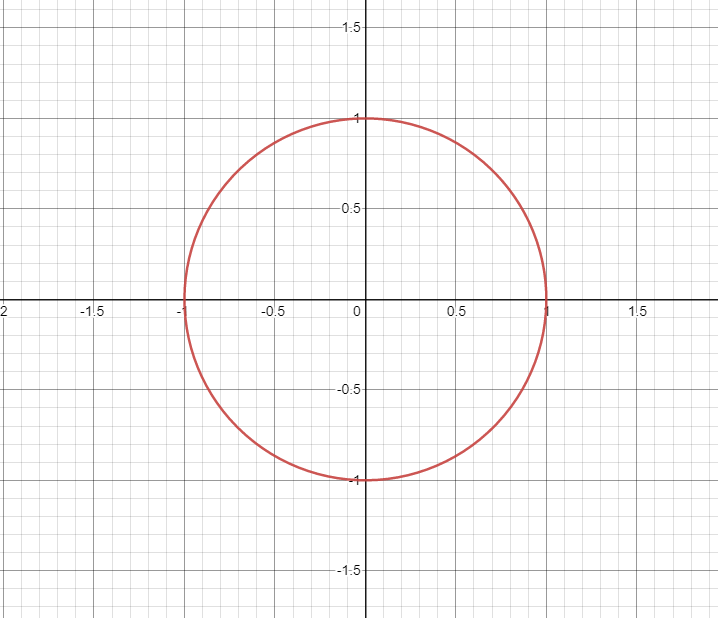Using desmos, I have graphed a unit circle using parametric coordinates, (a cosθ, a sinθ)

Similarly you can locate a point using a circle. For example, if I need to find the point (0.5,0.855), I put the angle corresponding to 0.5 in cos and 0.855 in sin (which is pi/3 radian or 60⁰) and I get the point.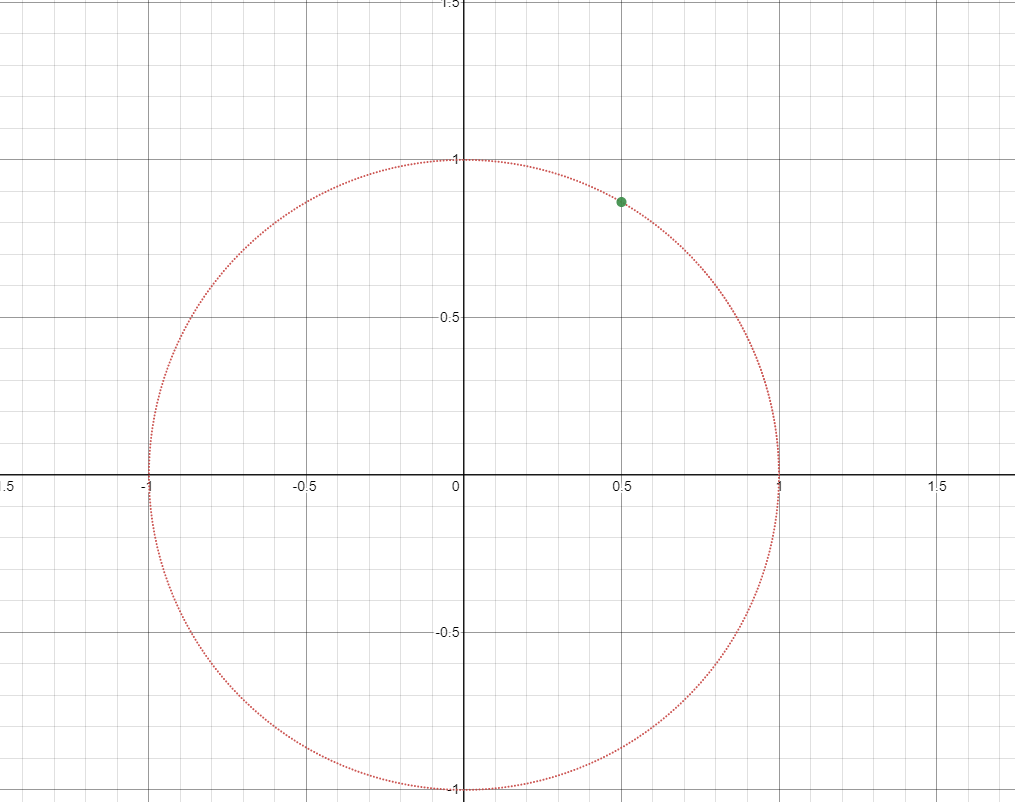a point on the unit circle can be specified using different angles for different values of cos and sin.

Similarly, you can graph complex numbers using the y axis as the imaginary part and the x axis as the real part. The point (x,y) in this plane (called the Gaussian plane) represents (x+iy).

# De Moivre’s Theorem

For De Moivre’s theorem to work, you need to know the representation of (a+bi) in the polar system of coordinates. We follow simple steps to do this.

First, square the real part and the real coefficient of i, add them and take the square root. Then multiply and divide by this factor.

(a+bi)= √(a²+b²)(a+bi)/√(a²+b²)

Now divide inside the bracket

(a+bi)= √(a²+b²)(a/√(a²+b²)+bi/√(a²+b²))

now find an angle whose values in cos and sin give a/√(a²+b²)&b /√(a²+b²) respectively. Let us call this angle θ.

(a+bi)= √(a²+b²)(cosθ+isinθ)

or

(a+bi)= √(a²+b²)(cisθ)

where cisθ represents (cosθ+isinθ). Here we have completed out conversion into polar form. Now we return to De Moivre’s Theorem.

It is important to note that θ Must be an angle in radians,

De Moivre’s Theorem states that if a complex number is in polar form, and raised to a power, either of these rules apply:-

1)(cos θ+ i sin θ)^n = cos(nθ) + i sin(nθ)

2) (cos θ+ i sin θ)^(p/q) = cos((p/q)θ) + i sin((p/q)θ)

Now there is an extension of the second rule, which we will be using and that is:

3) (cos θ+ i sin θ)^(p/q) = cos((2kπ+pθ/q)) + i sin((2kπ+pθ/q))

Here the number of solutions = q
and we give values of k starting with 0 and upto q-1, that is the set of values k can take is:
k ∈ {0,1,2,3,4,……,q-1}

# The Math

Now that we have understood De Moivre’s Rules, lets get into the action.

Let there be a complex number z(in the form of a+ib, remember that real numbers are complex numbers as well, because if b=0, there is no imaginary part) which is in the form of (cosθ+isinθ) in polar form, such that z-1³=0

Now we do some simple algebra to get:

Taking the cube root on both sides:

Now we convert z to the polar form.

Now we need to find a θ such that cos θ=1, and sin θ=0. What angle could that be? That’s right, its 0⁰!.

Let us now apply the extension we used in de Moivre’s Second rule:

Since p0=0, we get:

Now let us start thinking about the solution set of k:

k ∈ {0,1,2} (as q=3)

putting k=0, we get:1!

putting k=1, we get: -1/2 +√3i/2!

We call this root of unity as ω and ω=-1/2 +√3i/2

Putting k=2, we get: -1/2 -√3i/2!

We call this root of unity ω² as the if we do ω* ω, we get -1/2 -√3i/2.

There we have it, we have found out 3 cube roots of 1: namely 1, ω and ω²!

# Can we find the nth root of 1 using this method?

Yes! Yes we can! All we need to do is put q=n and you are good to go! Keep changing values of k and finding out different roots.

here, choose values of k from k ∈{0,1,2,3,4,……,n-1}. As an exercise, you can try to find out the values of fourth roots of unity using this method.

Let the numbers which are fourth root of unity be z, then,
z ∈ {1,-1,i,-i}
(given here you so you can check it)

This is it for this article! I hope you enjoyed reading this as much as i enjoyed writing it! Have good day ahead and I will see you soon!

# Bibliography

Credits for the images:
Charts on polar coordinates: desmos.com
First de Moivre’s Rule: calcworkshop.com

The equations I inserted in black and white were thanks to the help of mathbot latex on discord.

--

--

--

## More from Math Simplified

Simplified is a publication aiming at making mathematics accessible and enjoyable.

## Probability theory: Binomial distribution## Probability, Relativity and Pascal’s Triangle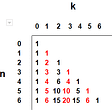## Leonhard Euler , Me and The Magic House Trilogy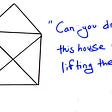## Towards a simple theorem prover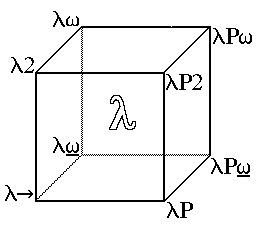## 5+ Reasons I Fell in Love With Math — Part I## Visualizing Eigenvalues and Eigenvectors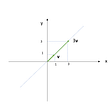## System of linear equations | Linear Algebra | Part 4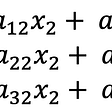## Depth first and breadth first search on trees in Javascript## veer vishal dubey

I like coding, math, physics, anime and cars.

## A Mathematical Proof that 2+2≠4 and 0.99…≠1 (Really!)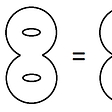## Why dividing by zero is undefined? — A simple explanation## An Elegant Exact Explicit Formula for Riemann Zeta Zero-Counting Function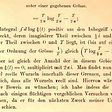## A List Of Geometry Problems